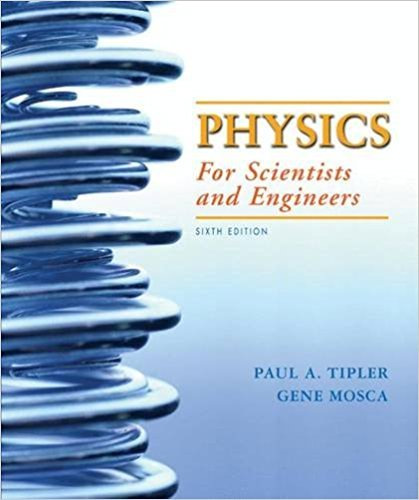×
Log in to StudySoup
Get Full Access to Physics For Scientists And Engineers, - 6 Edition - Chapter 30 - Problem 6
Join StudySoup for FREE
Get Full Access to Physics For Scientists And Engineers, - 6 Edition - Chapter 30 - Problem 6

Already have an account? Login here
×
Reset your password

# The detection of radio waves can be accomplished withISBN: 9781429201247 201

## Solution for problem 6 Chapter 30

Physics for Scientists and Engineers, | 6th Edition

• Textbook Solutions
• 2901 Step-by-step solutions solved by professors and subject experts
• Get 24/7 help from StudySoup virtual teaching assistantsPhysics for Scientists and Engineers, | 6th Edition

4 5 1 382 Reviews
12
3
Problem 6

The detection of radio waves can be accomplished with either an electric dipole antenna or a loop antenna. True or false: (a) The electric dipole antenna works according to the Faradays law. (b) If a linearly polarized radio wave is approaching you head on such that its electric field oscillates vertically, to best detect this wave the normal to a loop antennas plane should be oriented so that it points either right or left. (c) If a linearly polarized radio wave is approaching you such that its electric field oscillates in a horizontal plane, to best detect this wave using a dipole antenna the antenna should be oriented vertically

Step-by-Step Solution:
Step 1 of 3

Thomas 1 Trigonometry Example: Pythagorean Theorem: Scalars & Vectors Scalar quantities are just a number 1. Examples: a. Temperature b. Height c. Mass 2. Note that scaler quantities exclude physical quantities Vector Quantities 1. Vectors are described by magnitude and direction arrows. a. The arrows indicate the direction of the vector. b. Vectors can be used to describe forces, acceleration, and velocity. c. R vectors is proportional to the magnitude of the vector. Vector Addition 1. Consider magnitude and direction while using these. 2. All vectors as seen on a diagram should

Step 2 of 3

Step 3 of 3

##### ISBN: 9781429201247

The answer to “The detection of radio waves can be accomplished with either an electric dipole antenna or a loop antenna. True or false: (a) The electric dipole antenna works according to the Faradays law. (b) If a linearly polarized radio wave is approaching you head on such that its electric field oscillates vertically, to best detect this wave the normal to a loop antennas plane should be oriented so that it points either right or left. (c) If a linearly polarized radio wave is approaching you such that its electric field oscillates in a horizontal plane, to best detect this wave using a dipole antenna the antenna should be oriented vertically” is broken down into a number of easy to follow steps, and 109 words. Since the solution to 6 from 30 chapter was answered, more than 296 students have viewed the full step-by-step answer. This textbook survival guide was created for the textbook: Physics for Scientists and Engineers,, edition: 6. This full solution covers the following key subjects: . This expansive textbook survival guide covers 42 chapters, and 3686 solutions. Physics for Scientists and Engineers, was written by and is associated to the ISBN: 9781429201247. The full step-by-step solution to problem: 6 from chapter: 30 was answered by , our top Physics solution expert on 01/02/18, 09:00PM.

Unlock Textbook Solution

Enter your email below to unlock your verified solution to:

The detection of radio waves can be accomplished with